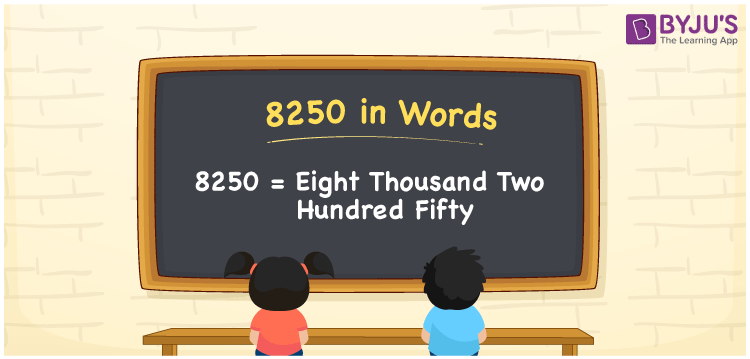# 8250 in Words

8250 in words is written as Eight thousand two hundred fifty. In both the International System of Numerals and the Indian System of Numerals, 8250 is written as Eight thousand two hundred fifty. The number 8250 is a Cardinal Number as it represents some quantity. For example, “the dress costs 8250 rupees.”

 8250 in Words Eight thousand two hundred fifty Eight thousand two hundred fifty in Number 8250

## 8250 in English Words

8250 in English words is read as “Eight thousand two hundred fifty.”## How to Write 8250 in Words?

To write 8250 in words, we shall use the place value chart. In the place value chart, put 8 in the thousands, 2 in the hundreds, 5 in the tens and 0 in the ones, respectively. Let us make a place value chart to write the number 8250 in words.

 Thousands Hundreds Tens Ones 8 2 5 0

Thus, we can write the expanded form as

8 × Thousand + 2 × Hundred + 5 × Ten + 0 × One

= 8 × 1000 + 2 × 100 + 5 × 10 + 0 × 1

= 8000 + 200 + 50 + 0

= 8250

= Eight thousand two hundred fifty.

8250 is a natural number, the successor of 8249 and the predecessor of 8251.

8250 in words – Eight thousand two hundred fifty

• Is 8250 an odd number? – No
• Is 8250 an even number? – Yes
• Is 8250 a perfect square number? – No
• Is 8250 a perfect cube number? – No
• Is 8250 a prime number? – No
• Is 8250 a composite number? – Yes

## Frequently Asked Questions on 8250 in Words

Q1

### How to write 8250 in words?

8250 in words is written as Eight thousand two hundred fifty.
Q2

### How to write 8250 in words in the International and Indian System of Numerals?

In both, the system of numerals, 8250 in words, is written as Eight thousand two hundred fifty.
Q3

### How to write 8250 in a place value chart?

In the place value chart, write 8 in the thousands, 2 in the hundreds, 5 in the tens and 0 in the ones, respectively.